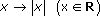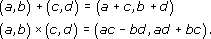## Question 1

Draw a line of symmetry on each of the shapes below.

There is another kind of symmetry which is often used in designs. It can be seen, for instance, in a car wheel trim.Look at the trim on the left. It does not have line symmetry but
Author(s): The Open University

Look at the shapes below. The symmetry of the shape on the left and its relationship to the shape on the right can be thought of in two ways:

• Fold the left-hand shape along the central line. Then one side lies exactly on top of the other, and gives the shape on the right.

• Imagine a mirror placed along the central dotted line. The reflection in the mirror gives the other half of the shape.

Author(s): The Open University

Drawing circles freehand often produces very uncircle-like shapes! If you need a reasonable circle, you could draw round a circular object, but if you need to draw an accurate circle with a particular radius, you will need a pair of compasses and a ruler. Using the ruler, set the distance between the point of the compasses and the tip of the pencil at the desired radius; place the point on the paper at the position where you want the centre of the circle to be and carefully rotate the compass
Author(s): The Open UniversityA quadrilateral is a shape with four straight sides.
Author(s): The Open University

This section deals with the simplest geometric shapes and their symmetries. All of the shapes are two-dimensional – hence they can be drawn accurately on paper.

Simple geometric shapes are studied in mathematics partly because they are used in thousands of practical applications. For instance, triangles occur in bridges, pylons and, more mundanely, in folding chairs; rectangles occur in windows, cinema screens and sheets of paper; while circles are an essential part of wheels, gears a
Author(s): The Open University

## Question 1

Find α and β in each of the diagrams below.

[Image_Link]https://www.open.edu/openlearn/ocw/pluginfile.php/94675/mod_oucontent/
Author(s): The Open University

Other pairs of equal angles can be identified in Example 5. These pairs of angles occur in a Z-shape, as indicated by the solid line in the diagram below. Such angles are called alternate angles.

[Image_Link]https://www.open.edu/openlearn/o
Author(s): The Open University

Look at the line l, which cuts two parallel lines m and n.If you trace the lines at one of the intersections in the diagram below and place them over the lines at the other interse
Author(s): The Open University

Two straight lines that do not intersect, no matter how far they are extended, are said to be parallel. Arrows are used to indicate parallel lines.Author(s): The Open University

## Question 1

Find all the remaining angles in each of the diagrams below.

[Image_Link]https://www.open.edu/openlearn/ocw/pluginfile.php/94675/mod_oucontent/
Author(s): The Open University

When two straight lines cross, they form four angles. In the diagram below, these angles are labelled α, β, θ and φ and referred to as alpha, beta, theta and phi. The angles opposite each other are equal. They are called vertically opposite angles. Here α and β are a pair of vertically opposite angles, as are θ and φ. Although such angles are called ‘vertically opposite’, they do not need to be vertically above and bel
Author(s): The Open University

## Question 1

A company carried out a survey, recording how staff in a particular office spent their working time. The table shows the average number of minutes spent in each hour on various activities.

Author(s): The Open University

## Question 1

Calculate all the angles at the centres of these objects.

You will no doubt recall that in real analysis extensive use is made of the modulus function. It gives us a way of measuring the “closeness” of two numbers, which we exploit in writing expressi
Author(s): The Open University

In this section we have seen a correspondence between complex numbers and points in the plane using Cartesian coordinates; the real part of the complex number is represented on the real axis (“horizontal”) and the imaginary part on the imaginary axis (“vertical”). We can also use polar coordinates (r,θ) in which case r, the modulus of a non-zero complex number z is positive and θ is an argument of z, defined only to within an additive intege
Author(s): The Open University

We have seen in Section 2.2 that the complex number system is obtained by defining arithmetic operations on the set R × R. We also know that elements of R × R can be represented as points in a plane. It seems reasonable to ask what insight can be obtained by representing complex numbers as
Author(s): The Open University

In this section we have seen that the complex number system is the set R × R together with the operations + and × defined byFrom this, one can justify the performance of ordinary algebraic operations on expressions of the form
Author(s): The Open University

You have almost certainly met complex numbers before, but you may well not have had much experience in manipulating them. In this course we provide you with an opportunity to gain confidence in working with complex numbers by working through a number of suitable problems.

Perhaps the most striking difference between real numbers and complex numbers is the fact that complex numbers have a two-dimensional character, arising from our definition of a complex number as an ordered pair of
Author(s): The Open UniversityLearning outcomes

After studying this course, you should be able to:

• perform basic algebraic manipulation with complex numbers

• understand the geometric interpretation of complex numbers

• know methods of finding the nth roots of complex numbers and the solutions of simple polynomial equations.

Author(s): The Open University